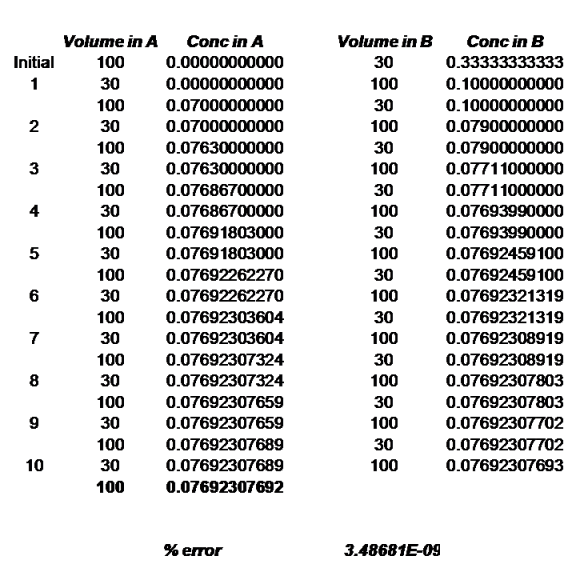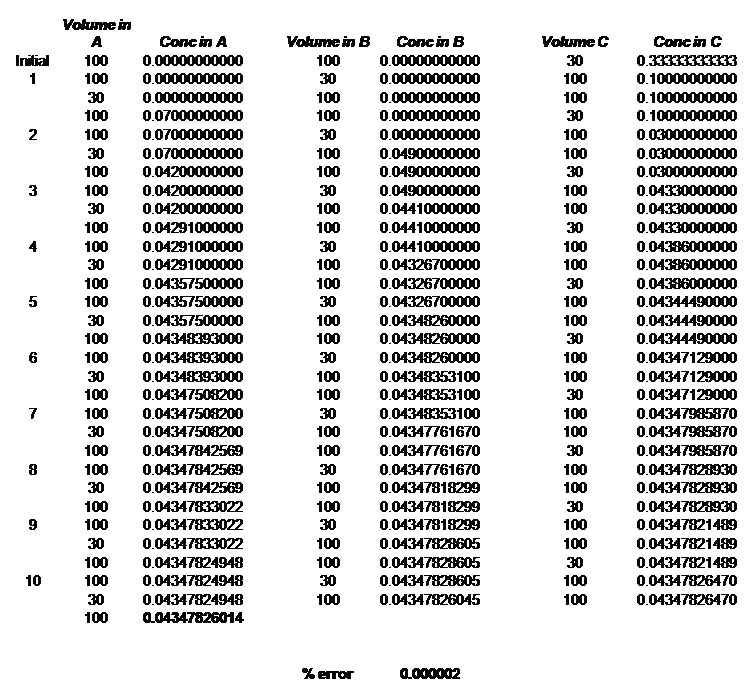#### You may also like### Golden Thoughts

Rectangle PQRS has X and Y on the edges. Triangles PQY, YRX and XSP have equal areas. Prove X and Y divide the sides of PQRS in the golden ratio.### At a Glance

The area of a regular pentagon looks about twice as a big as the pentangle star drawn within it. Is it?### Contact

A circular plate rolls in contact with the sides of a rectangular tray. How much of its circumference comes into contact with the sides of the tray when it rolls around one circuit?

# Exact Dilutions

##### Age 14 to 16Challenge Level

in this problem there are two different mathematical operations which can be used: You can dilute a dilution, which corresponds to multiplying fractions; you can mix two dilutions, which corresponds to averaging two fractions.

Part 1:

1) To create a solution of $\frac{1}{3}$, pipette 10ml of solution then 2 x 10ml of water into the 100ml beaker.

2) To create a solution of $\frac{1}{7}$, pipette 10ml of solution followed by 6 x10ml of water into the 100ml beaker.

3) To create a solution of $\frac{1}{18}$ first create a solution of $\frac{1}{9}$ by pipetting 10ml of solution followed by 8 x 10ml of water into the 100ml beaker. Then, pippette 10ml of this solution into another beaker, followed by 10ml of water to give the required concentration.

4) To create a solution of $\frac{5}{12}$ first create a solution of $\frac{5}{6}$ by pipetting 5 x 10ml of solution followed by 10ml of water into the 100ml beaker. Then pipette 10ml of this solution into the second 100ml beaker, followed again by 10ml of water.

5) To create a solution of $\frac{29}{35}$ first note that $\frac{29}{35}=\frac{2}{5}+\frac{3}{7}$. This means that $\frac{29}{35}$ is the average of $\frac{4}{5}$ and $\frac{6}{7}$, so our strategy is to mix equal amounts of these solutions. First make a $\frac{4}{5}$ solution in beaker A by pipetting 4 x 10ml of solution followed by 10ml of water. Then make a $\frac{6}{7}$ solution in beaker B by pipetting 6 x 10ml of solution followed by 10ml of water. Finally, pipette 10ml of the solution in beaker A and 10ml of the solution in beaker B into beaker C, creating 20ml of a $\frac{29}{35}$ solution.

6) To create a solution of $\frac{71}{882}$ requires a few steps. First, create a $\frac{4}{7}$ solution by pipetting 4 x 10ml into the beaker, followed by 3 x 10ml water. Then pippette out 10ml of this into another beaker, followed by 6 x 10ml of water, to give a solution of $\frac{4}{49}$. Discard the original $\frac{4}{7}$ solution and create a $\frac{5}{7}$ solution by pipetting 5 x 10ml solution then 2 x 10ml water. Pipette 10ml of this out into the third beaker, then add 8 x 10 ml of water to give a $\frac{5}{63}$ solution. Now, mix equal amounts of this solution with the $\frac{4}{49}$ solution to give the required $\frac{71}{882}$.

NB there is more than one way to make each solution!

Part 2:

The concentrations of $\frac{1}{2}$ to $\frac{1}{10}$ are trivially created by adding 10ml of solution, followed by (n-1) x 10ml of solution, where n is the denominator of the concentration to be created.

For dilutions less than this, it is not possible to create those which have a denominator which is a PRIME number $p$. Indeed, it is not possible to multiply together two numbers (i.e dilute a dilution) to give this number, and averaging two fractions (i.e mixing two existing dilutions) with denominators which aren't divisible by $p$ gives a fraction whose denominator is not divisible by $p$.

To create the dilutions which are non-prime involves diluting an already made solution. For example, creating $\frac{1}{18}$ involves taking the $\frac{1}{9}$ solution and mixing it in a 1:1 ratio with water.

To see whether it is possible to create a dilution of $\frac{1}{362880}$ it is best to express 362880 as a produce of primes, and to see whether any of these primes are greater than 10. If so, then the solution cannot be made, but if not, then it can be made readily.

$362880 = 2^9 \times 5 \times 7 \times 9^2$. Thus it is possible to make!

Part 3:

Common sense tells us that by mixing a solution of concentration $c_A$ and $c_B$ we will obtain a solution with an intermediate concentration. It can be seen that we will not obtain a more concentrated solution than either, since there are insufficient moles being added for this to be possible! Also, to get a more dilute solution, water would need to be added.

This can be proved as follows:

Suppose I mix $a$ units of $A$ with $b$ units of $B$.

Using the general concentration formula I get $$\frac{ac_A+bc_B}{a+b} = c_C$$

I can rearrange this to give $$c_A = \frac{(a+b)c_C-bc_B}{a}$$

Next note that $c_A> c_B$, so I have $$\frac{(a+b)c_C-bc_B}{a}> c_B$$

This reduces to $c_C> c_B$ (using positivity of and b, of course)

Below is a spreadsheet for the creation of the $\frac{1}{13}$ dilution. Notice how tiny the error is after only 10 repeats of steps 3 and 4!!!You might be wondering how this actually works. Overall we have placed 10ml of solution among 120ml of water, which is the required concentration. We have then essentially mixed it all together as much as possible until the concentration is constant between both beakers, which will give an overall concentration of $\frac{1}{13}$.

To get a concentration of $\frac{1}{23}$ requires the use of the third beaker. Again, we must add 10ml of solution to 220ml of water. This can be facilitated by having beaker A and B full of water, and Beaker C having 10ml of solution and 20ml of water. B is used to fill C, and then A is used to fill B. After this C is used to fill A, and the cycle repeated. Below is a spreadsheet for 10 iterations: